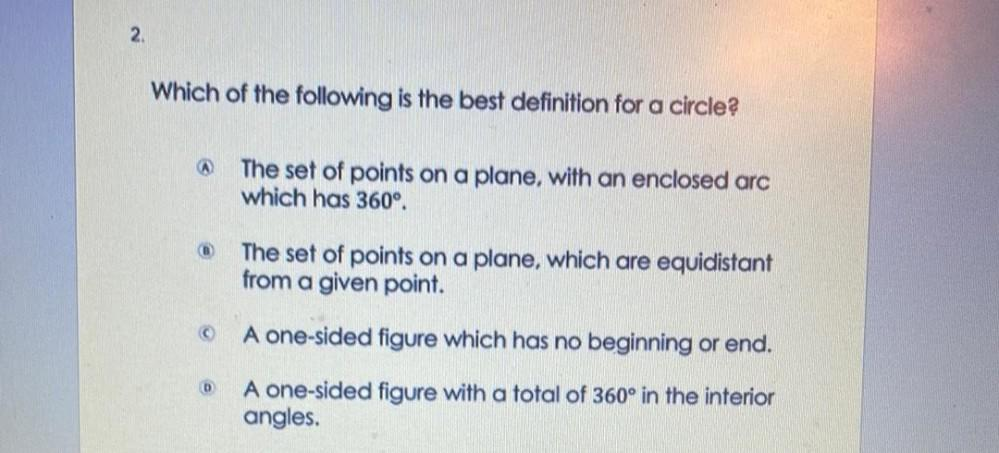Question:

# Which of the following is the best definition for a circle? a)The set of points on a plane, with an enclosed arc which has 360° b))The set of points on a plane, which are equidistant from a given poWhich of the following is the best definition for a circle? a)The set of points on a plane, with an enclosed arc which has 360° b))The set of points on a plane, which are equidistant from a given point. c)one-sided figure which has no beginning or end. D)A one-sided figure with a total of 360° in the interiorangles.